Courses
Courses for Kids
Free study material
Offline Centres
More

# 2-Digit Addition For Class 1Last updated date: 24th Nov 2023
Total views: 98.1k
Views today: 2.98k## Introduction

Addition with 2-digit numbers is adding two or more numbers with two digits. The result obtained is known as the sum of the two numbers.

To find a sum, an addend and an addend are added. The sum may also be used to refer to the total. Addition is one of the four basic operations of Mathematics, with the other three being subtraction, multiplication, and division. Addition can also be defined as the combination of multiple things together. It is impossible to live our daily lives without performing addition.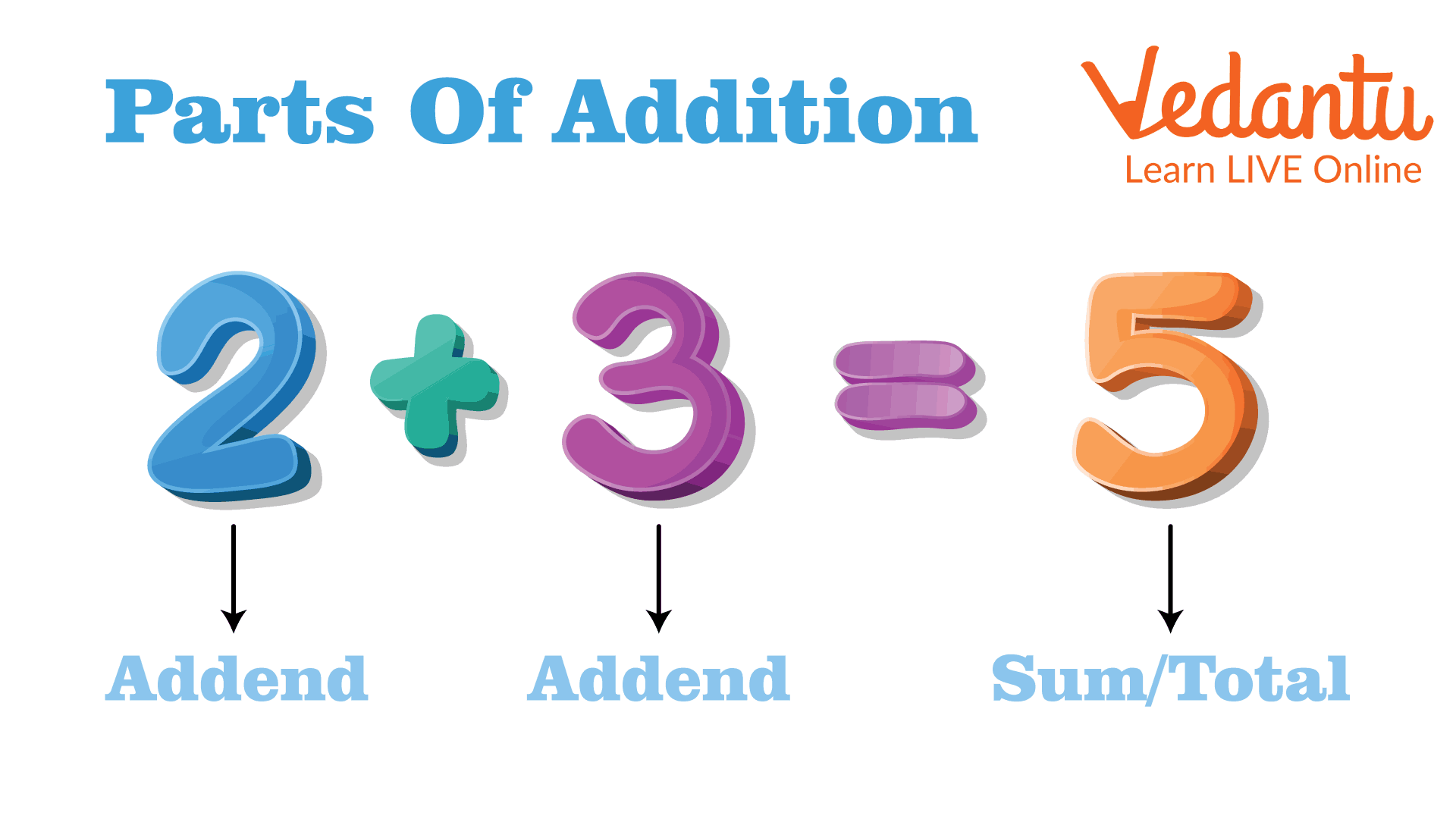There are four basic operations of arithmetic, i.e., addition, subtraction, multiplication, and division. Today, we will discuss addition or, be more precise, two-digit addition. It is the process of adding two or more numbers together, and the result obtained is known as the sum of the given numbers. Every arithmetic operation has its unique symbol. In addition, the sign is ‘+’.

## How Does Adding 2-Digit Numbers Work?

In addition, the place value of the numbers is given more importance. Here, the place value is expressed as ones and tens. To add 2 digits numbers, first, we need to arrange them column-wise according to their place values. Each digit is individually added. The answer is known as their total, and they are known as addends.

There are two methods for adding two digits. The first two are the "without regrouping" approach and the "regrouping" method, both of which are referred to as carrying forward.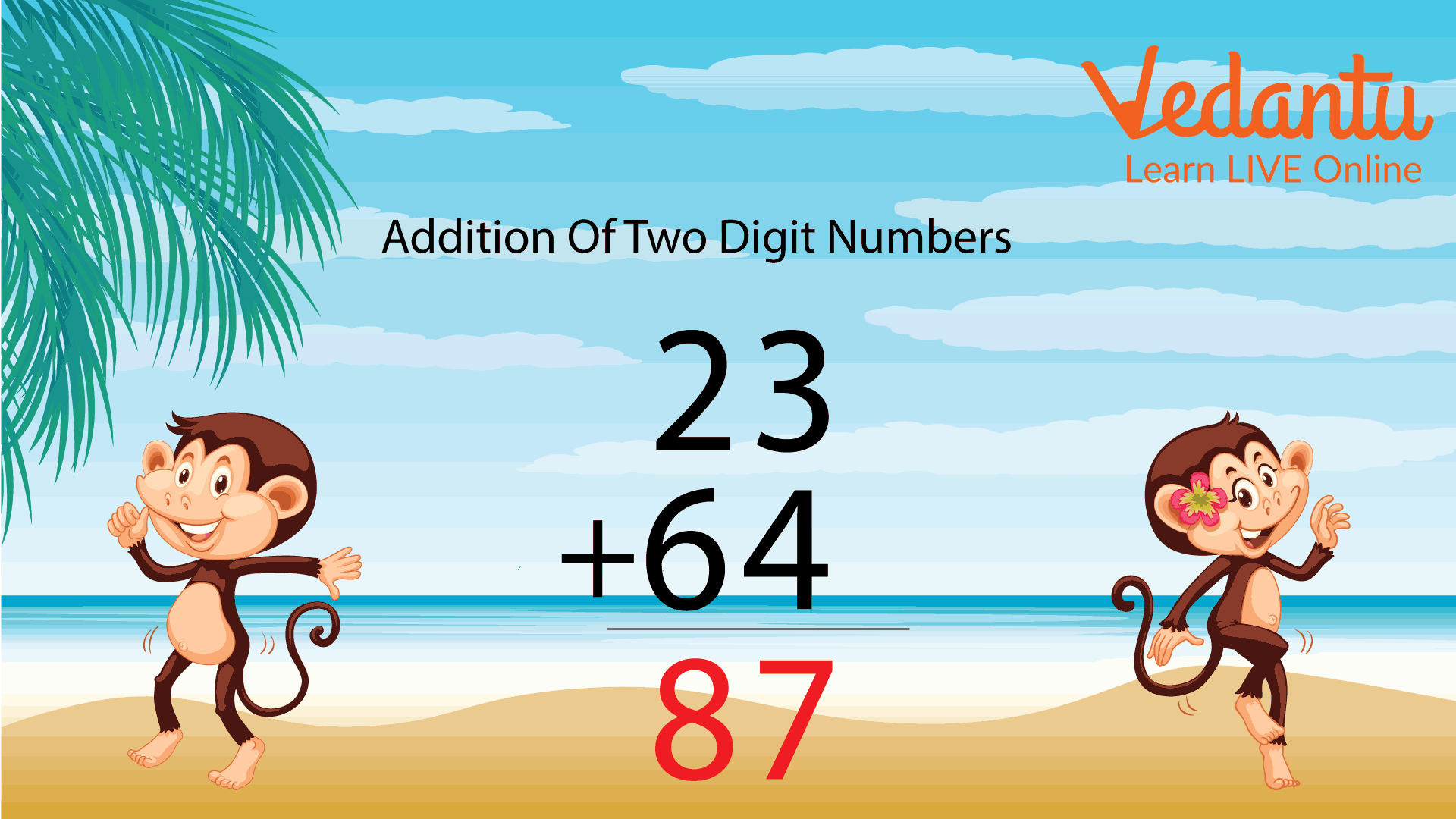Let us see some examples of two-digit addition:

Example 1:

Solution: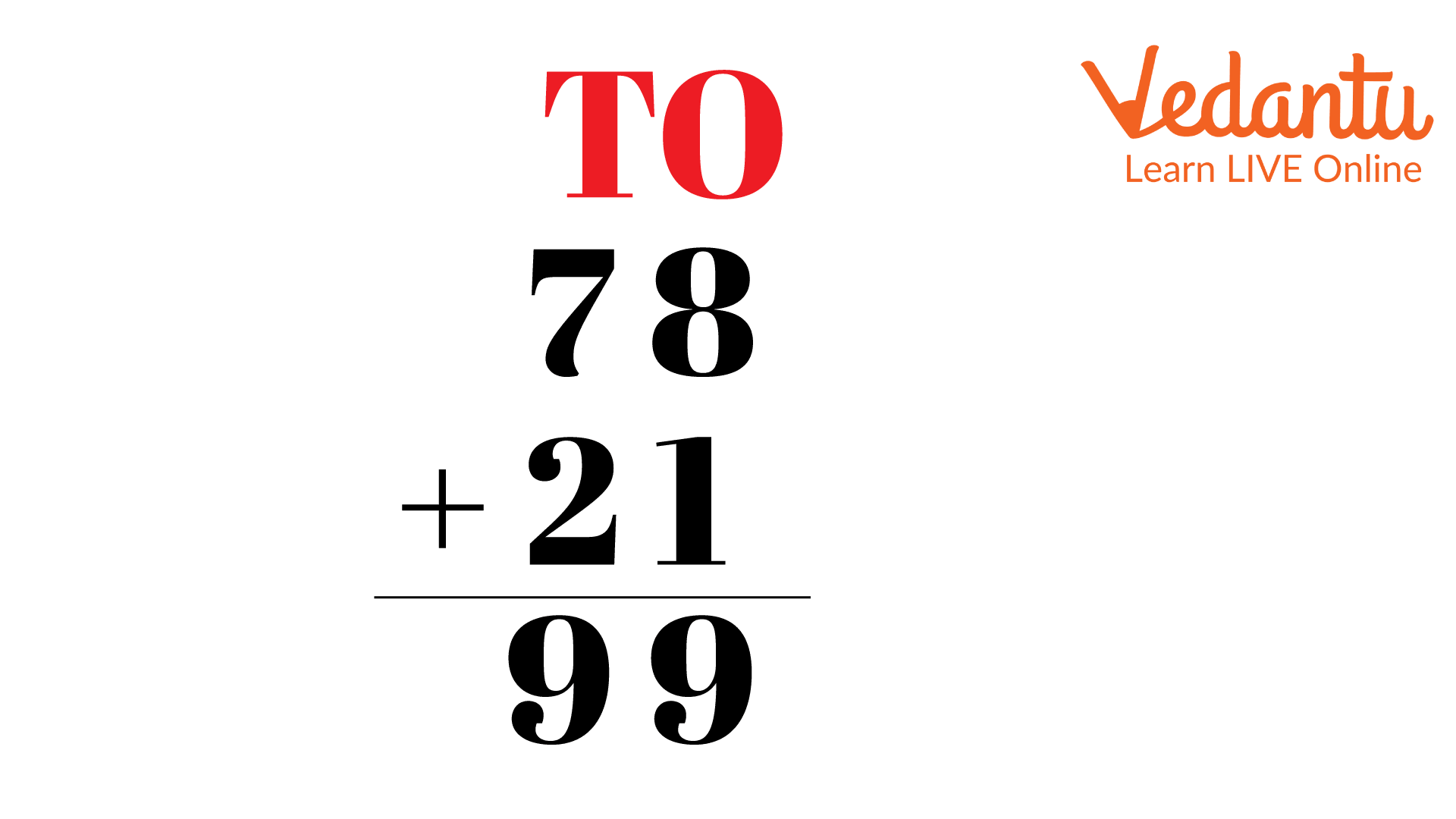$78 + 21$

Add the numbers of the ones columns: $8 + 1 = 9$

Add the numbers of the tens columns: $7 + 2 = 9$

Therefore, the result is 99.

Example 2:

Solution: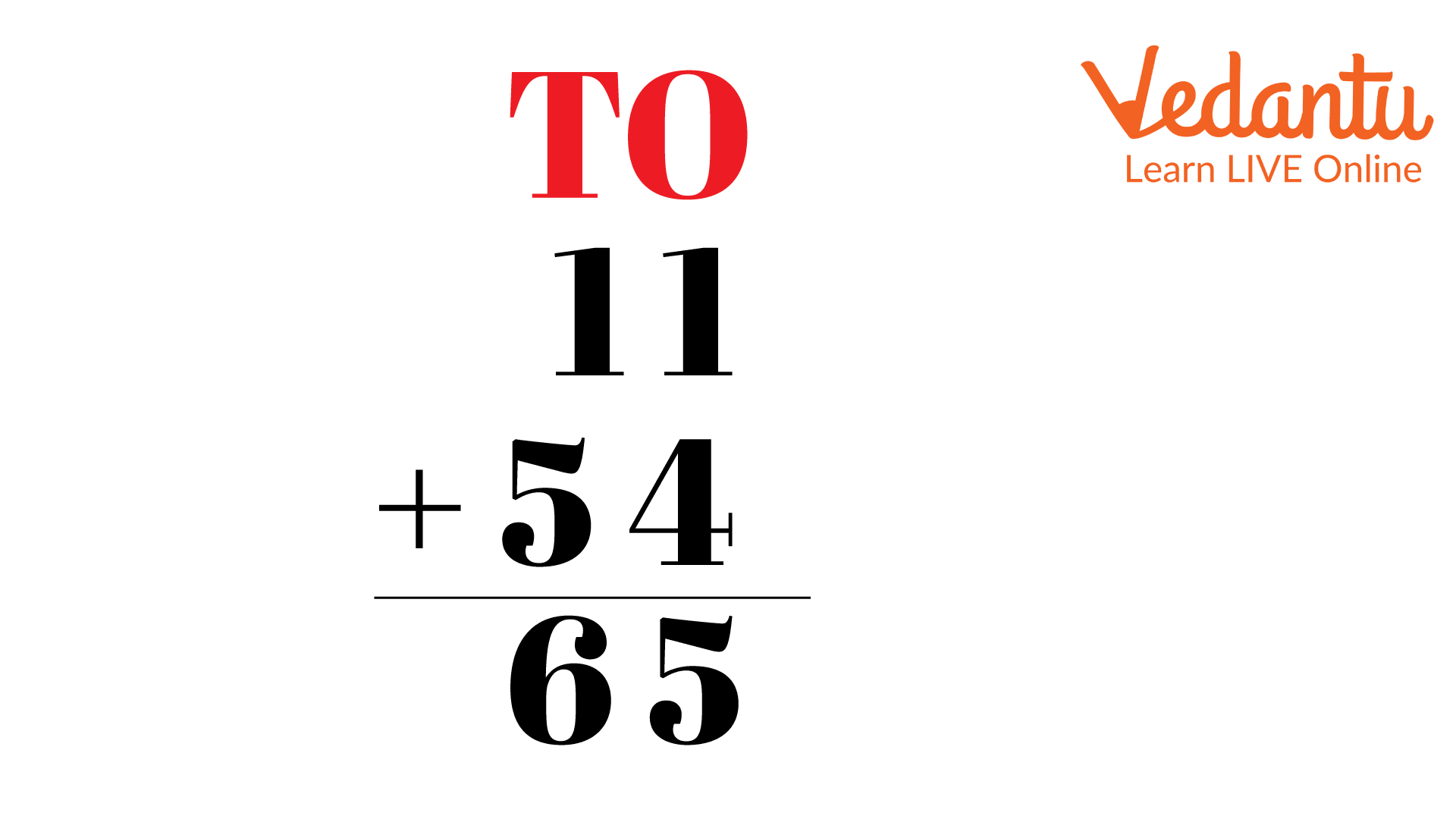$11 + 54$

Add the numbers of the ones columns: $1 + 4 = 5$

Add the numbers of the tens columns: $1 + 5 = 6$

Therefore, the result is 65.

Example 3:

Solution: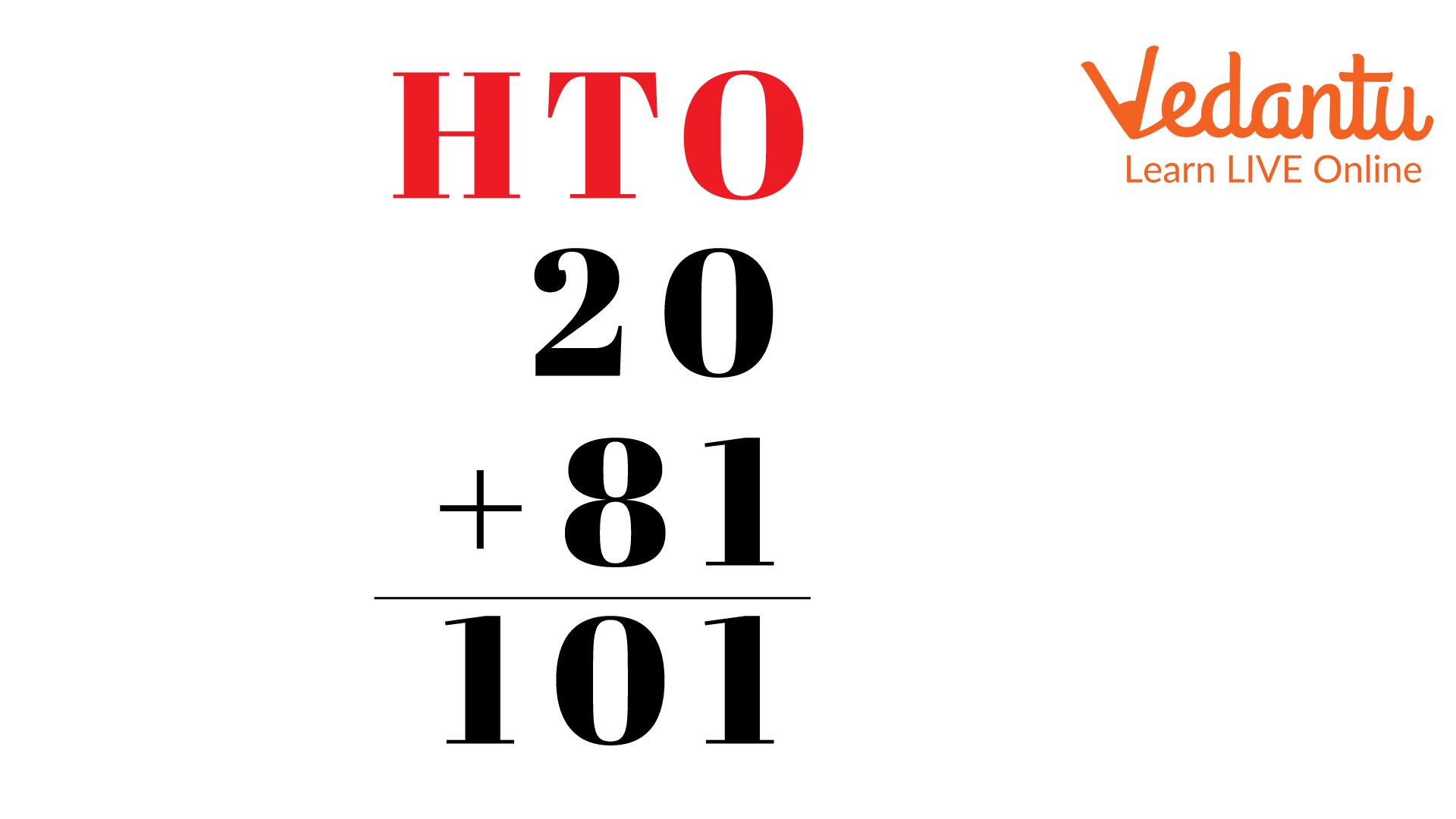$20 + 81$

Add the numbers of the ones columns: $0 + 1 = 1$

Add the numbers of the tens columns: $2 + 8 = 10$

Therefore, the result is 101.

Now, let us solve some addition worksheets for grade 1 that are given below:

Example 1:

Add the 2-digit numbers: 23 and 16.

Solution: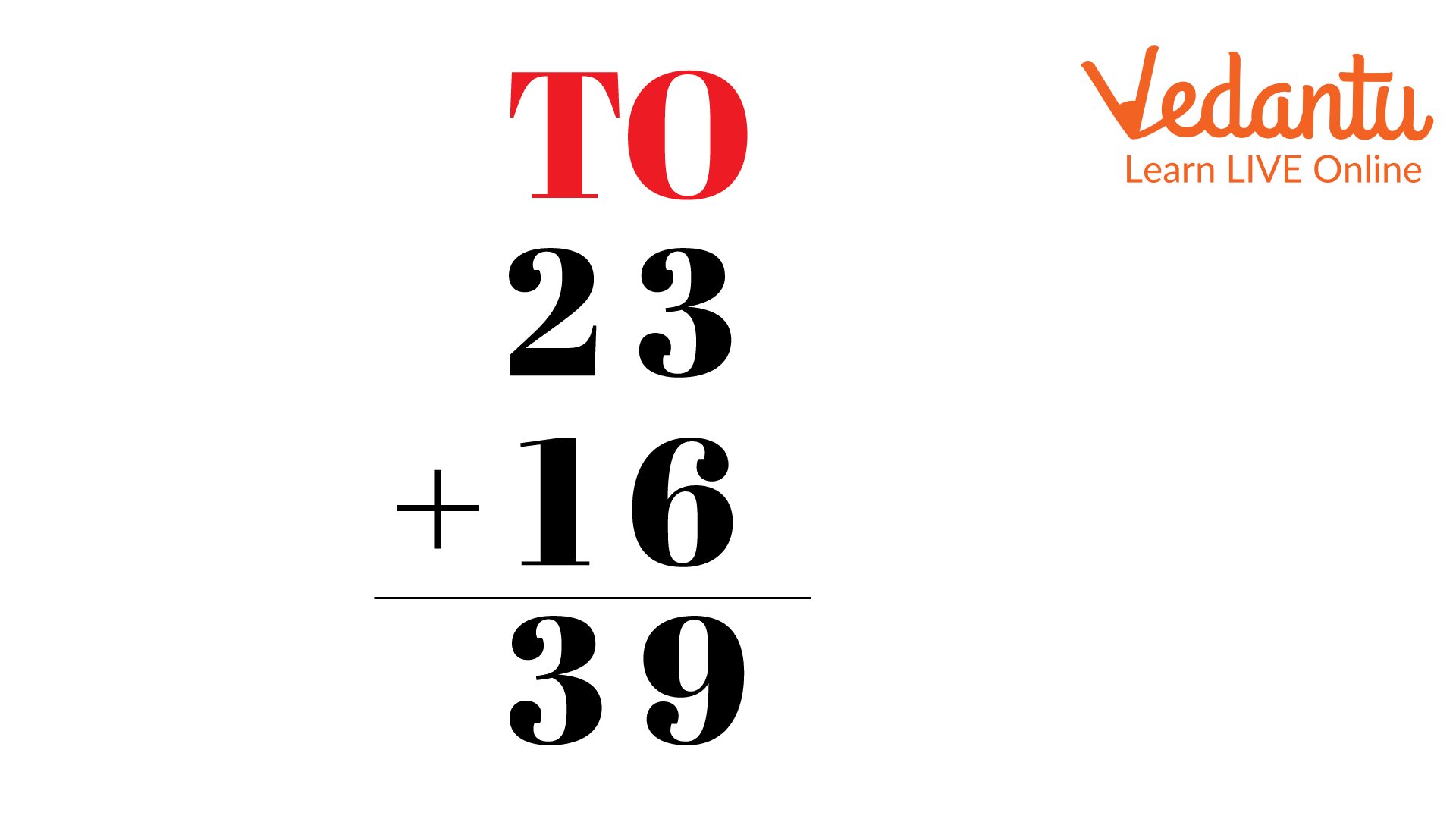$23 + 16$

Numbers in the ones columns: $3{\rm{ }} + {\rm{ }}6{\rm{ }} = {\rm{ }}9$

Numbers in the tens columns: $2{\rm{ }} + {\rm{ }}1{\rm{ }} = {\rm{ }}3$

Therefore, the result we obtained is $23{\rm{ }} + {\rm{ }}16{\rm{ }} = {\rm{ }}39$.

Example 2:

Add the 2-digit numbers: 40 and 28.

Solution: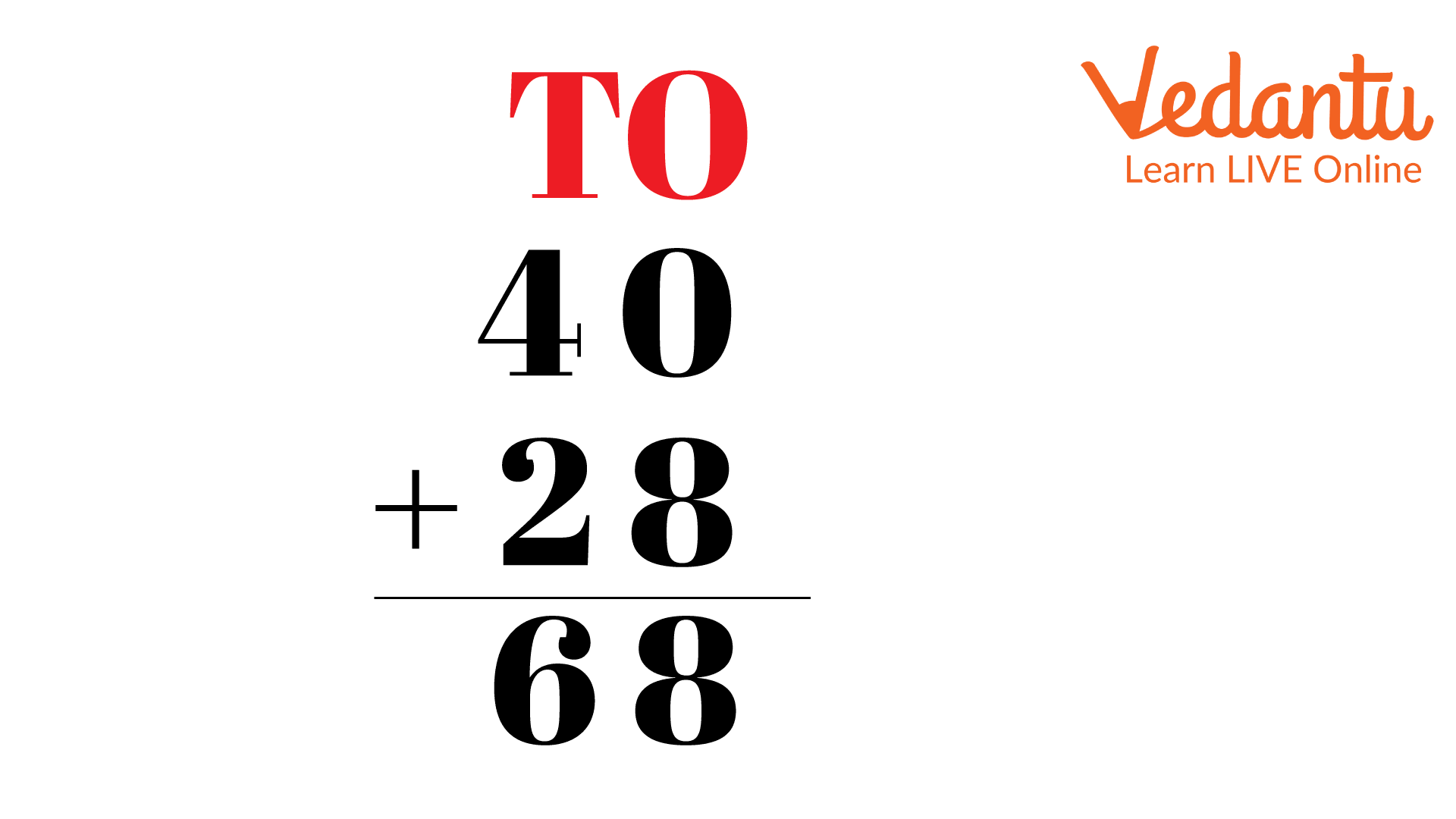$40 + 28$

Numbers in the ones columns: $0{\rm{ }} + {\rm{ }}8{\rm{ }} = {\rm{ }}8$

Numbers in the tens columns: $4{\rm{ }} + {\rm{ }}2{\rm{ }} = {\rm{ }}6$

Therefore, the result we obtained is $40{\rm{ }} + {\rm{ }}28{\rm{ }} = {\rm{ }}68$.

Example 3:

Add the 2-digit numbers: 62 and 52.

Solution: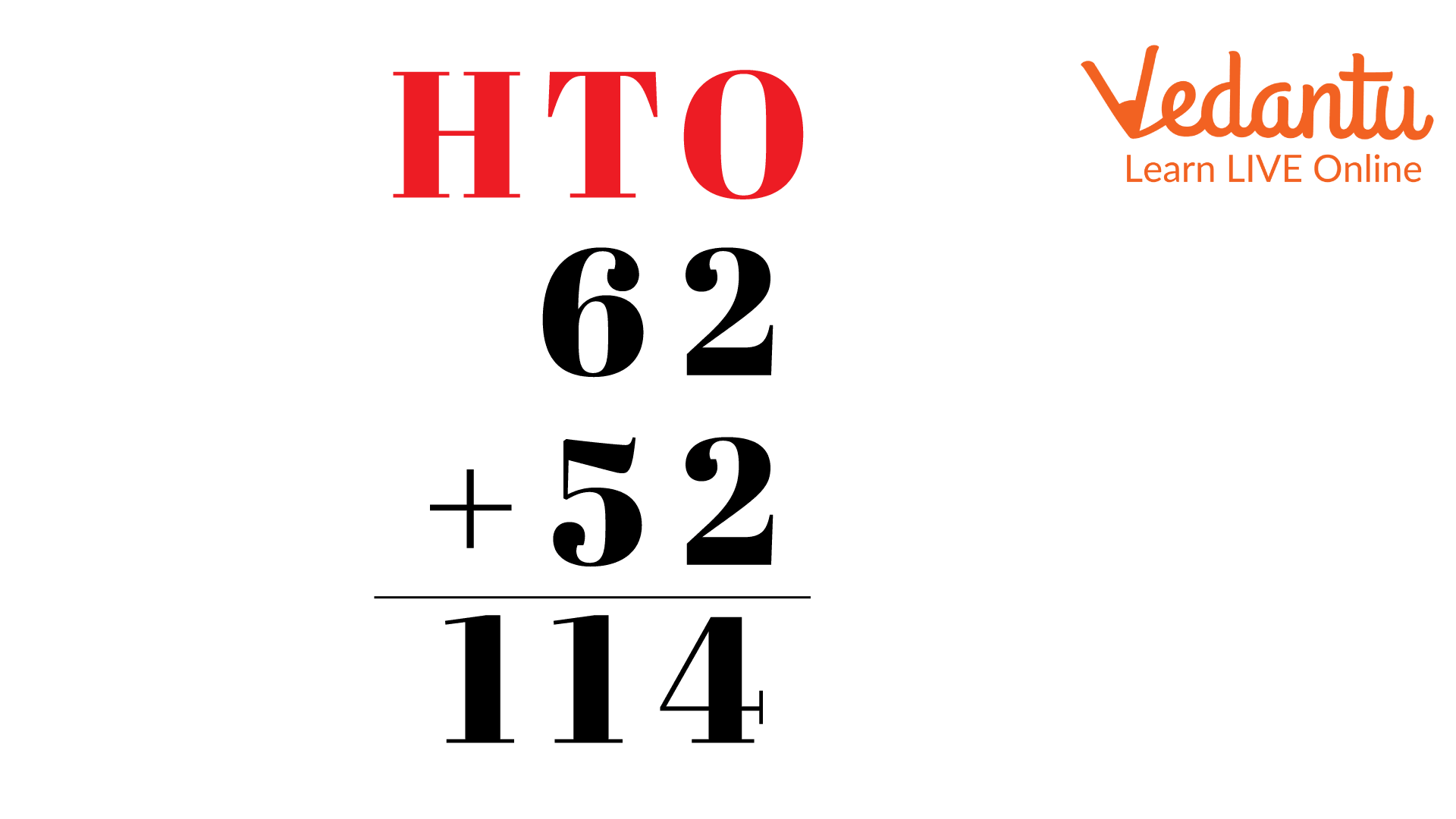$62 + 52$

Numbers in the ones columns: $2{\rm{ }} + {\rm{ }}2{\rm{ }} = {\rm{ }}4$

Numbers in the tens columns: $6{\rm{ }} + {\rm{ }}5{\rm{ }} = {\rm{ }}11$

Therefore, the result we obtained is $62{\rm{ }} + {\rm{ }}52{\rm{ }} = {\rm{ }}114$.

Example 4:

Add the 2-digit numbers: 43 and 14.

Solution: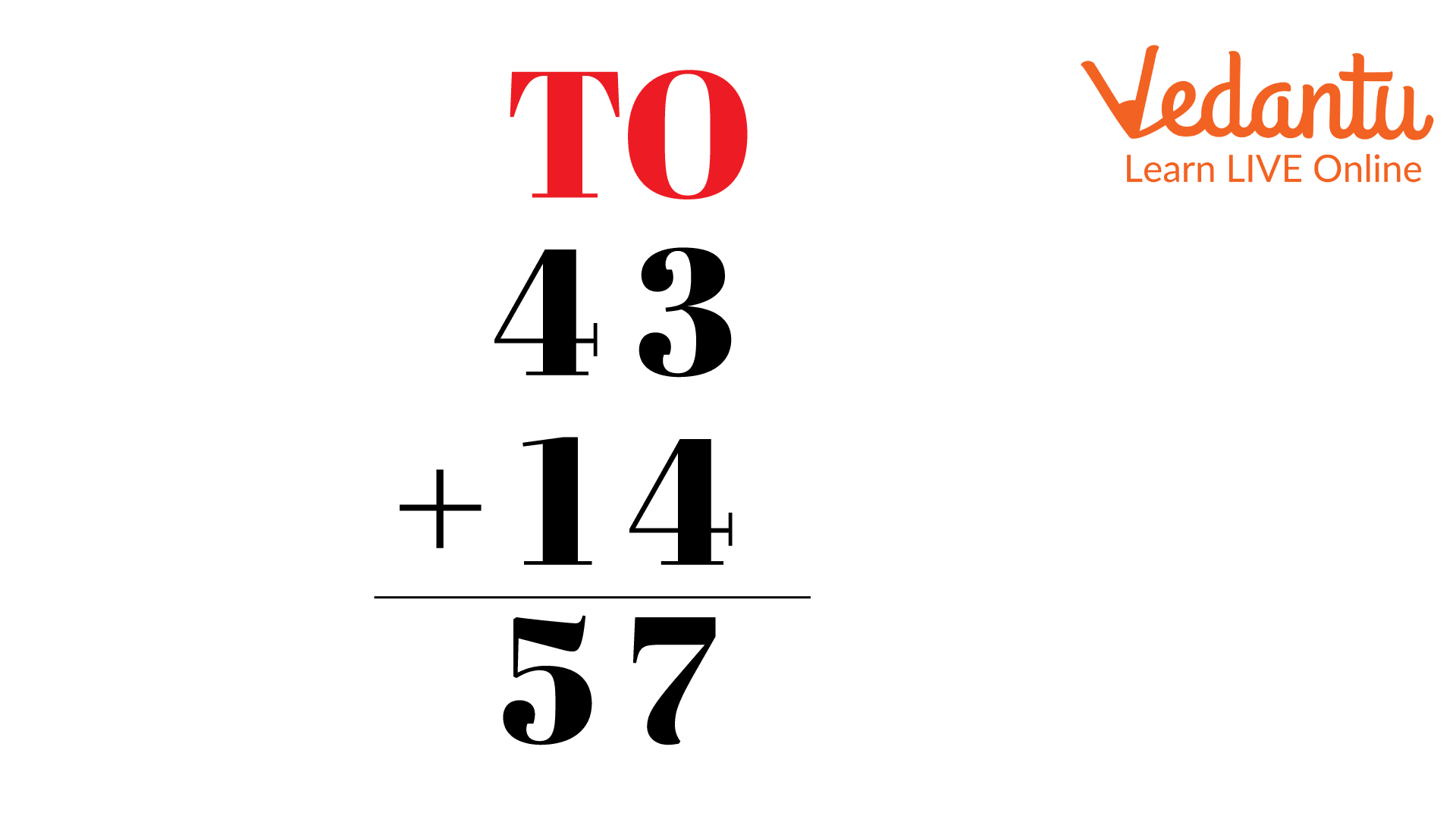$43 + 14$

Numbers in the ones columns: $3{\rm{ }} + {\rm{ }}4{\rm{ }} = {\rm{ }}7$

Numbers in the tens columns: $4{\rm{ }} + {\rm{ }}1{\rm{ }} = {\rm{ }}5$

Therefore, the result we obtained is $43{\rm{ }} + {\rm{ }}14{\rm{ }} = {\rm{ }}57$.

### Conclusion

The term Arithmetic Addition in mathematics refers to adding two or more numbers together for forming the total and the mathematics symbol or operator, which denotes that the addition is the + plus sign, for example - 8 + 8 = 16. The term Sum also denotes the same operation of addition.

## FAQs on 2-Digit Addition For Class 1

1: What does it mean when we say Sita and Sima have 20 apples altogether?

When we say Sita and Sima have 20 apples altogether, it means that if Sita has x apples, then Sima has a difference of 20 and x apples. On combining all those apples, they have 20 in total.

2: What does the idea of two-digit addition mean?

In two-digit addition, numerals are first placed according to their place value of ones and tens, and then they are added. Beginning with the one's column and working our way up to the tens column, we add from right to left. We get the total after adding the values from both columns.

3: What happens when we add something to 0?

We know that addition is a combination of two or more values. When we combine 2 and 1, it is the sum of the two values. Now, if we say Rita has 0 apples and Tina has 2 apples, and if we are asked to find the total number of apples both have. The answer will always be 2 apples, as Rita has 0, which means she doesn't have any apples, so the only apple being counted is the ones Tina has. Hence, we get the number itself whenever we add something to 0.